# Poly Decomp

## Mark Keil's Algorithm

### Algorithm

Without optimization tweaks, Keil's algorithm is actually quite straight-forward. A polygon can be decomposed into convex regions by simply eliminating all reflex vertices. Keil's algorithm works by examining every possible way to remove the reflexity of these vertices, and then takes the one that requires the fewest diagonals.

Pseudo-code:

```diags = decomp(poly) min, tmp : EdgeList ndiags : Integer for each reflex vertex i for every other vertex j if i can see j left = the polygon given by vertices i to j right = the polygon given by vertices j to i tmp = decomp(left) + decomp(right) if(tmp.size < ndiags) min = tmp ndiags = tmp.size min += the diagonal i to j return min ```

### Optimality

Since this algorithm tries all possible solutions and takes the best one, it is always optimal (produces a minimal number of convex regions) for the case without Steiner vertices.

### Complexity

By looking at the pseudo-code, we can see that there are two nested for loops that iterate over every vertex. The "can see" check also needs to loop over each edge to see if any of them are blocking the visibility. Then the algorithm recurses for each "half" of the polygon, on either side of the diagonal. This gives us the the familiar recurrence relation T(n) = 2*T(n/2) + O(n3), which would evaluate to O(n^3 log n). However, in a degenerate case, the polygon can be split unevenly, giving us T(n) = T(1) + T(n-1) + O(n3) = O(n4) worst case.

### Screenshots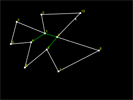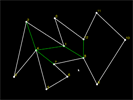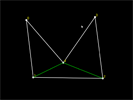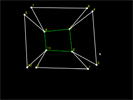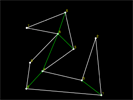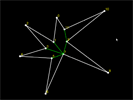```cmake . make ./conv```